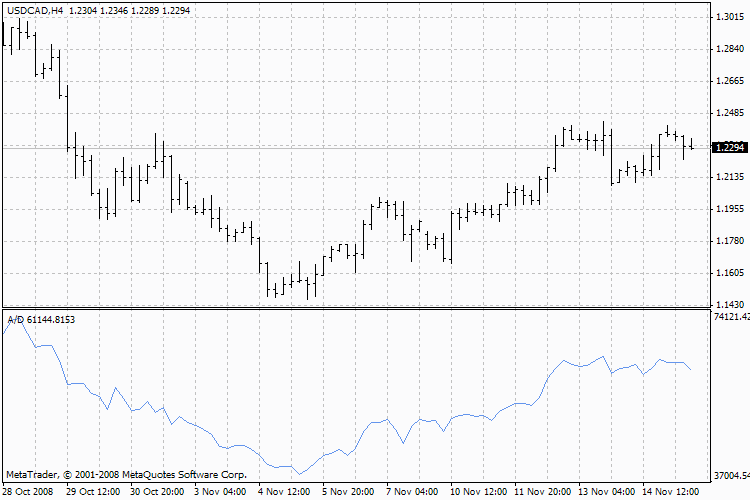Accumulation Distribution

Accumulation Distribution

29119
0
SHAREUsable across time frames, this indicator is based on price and volume, and can be used to confirm the validity of moves in the market.

Accumulation/Distribution Technical Indicator is determined by the changes in price and volume. The volume acts as a weighting coefficient at the change of price — the higher the coefficient (the volume) is, the greater the contribution of the price change (for this period of time) will be in the value of the indicator.n fact, this indicator is a variant of the more commonly used indicator On Balance Volume. They are both used to confirm price changes by means of measuring the respective volume of sales.

When the Accumulation/Distribution indicator grows, it means accumulation (buying) of a particular security, as the overwhelming share of the sales volume is related to an upward trend of prices. When the indicator drops, it means distribution (selling) of the security, as most of sales take place during the downward price movement.

Divergences between the Accumulation/Distribution indicator and the price of the security indicate the upcoming change of prices. As a rule, in case of such divergences, the price tendency moves in the direction in which the indicator moves. Thus, if the indicator is growing, and the price of the security is dropping, a turnaround of price should be expected.

Calculation

A certain share of the daily volume is added to or subtracted from the current accumulated value of the indicator. The nearer the closing price to the maximum price of the day is, the higher the added share will be. The nearer the closing price to the minimum price of the day is, the greater the subtracted share will be. If the closing price is exactly in between the maximum and minimum of the day, the indicator value remains unchanged.

A/D(i) =((CLOSE(i) – LOW(i)) – (HIGH(i) – CLOSE(i)) * VOLUME(i) / (HIGH(i) – LOW(i)) + A/D(i-1)

Where:
A/D(i) — importance of the Indicator of the Accumulation/Distribution for the current bar;
CLOSE(i) — the price of the closing the bar;
LOW(i) — the minimum price of the bar;
HIGH(i) — the maximum price of the bar;
VOLUME(i) — volume;
A/D(i-1) — importance of the Indicator of the Accumulation/Distribution for previous bar.

Accumulation Distribution is a Metatrader 4 (MT4) indicator and the essence of the forex indicator is to transform the accumulated history data.

Accumulation Distribution provides for an opportunity to detect various peculiarities and patterns in price dynamics which are invisible to the naked eye.

Based on this information, traders can assume further price movement and adjust their strategy accordingly.

How to install Accumulation Distribution.mq4?

• Copy Accumulation Distribution.mq4 to your Metatrader Directory / experts / indicators /
• Select Chart and Timeframe where you want to test your indicator
• Right click on Accumulation Distribution.mq4
• Attach to a chart
• Modify settings or press ok
• Indicator Accumulation Distribution.mq4 is available on your Chart

• Select the Chart where is the Indicator running in your Metatrader Client
• Right click into the Chart
• “Indicators list”
• Select the Indicator and delete